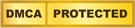# Improve Vectorization Performance using Intel Advanced Vector Extensions 512

Published Date
04 - Apr - 2017
| Last Updated
04 - Apr - 2017This article shows a simple example of a loop that was not vectorized by the Intel® C++ Compiler due to possible data dependencies, but which has now been vectorized using the Intel® Advanced Vector Extensions 512 instruction set on an Intel® Xeon Phi™ processor. We will explore why the compiler using this instruction set automatically recognizes the loop as vectorizable and will discuss some issues about the vectorization performance.

Introduction

When optimizing code, the first efforts should be focused on vectorization. The most fundamental way to efficiently utilize the resources in modern processors is to write code that can run in vector mode by taking advantage of special hardware like vector registers and SIMD (Single Instruction Multiple Data) instructions. Data parallelism in the algorithm/code is exploited in this stage of the optimization process.

Making the most of fine grain parallelism through vectorization will allow the performance of software applications to scale with the number of cores in the processor by using multithreading and multitasking. Efficient use of single-core resources will be critical in the overall performance of the multithreaded application, because of the multiplicative effect of vectorization and multithreading.

The new Intel® Xeon Phi™ processor features 512-bit wide vector registers. The new Intel® Advanced Vector Extensions 512 (Intel® AVX-512) instruction set architecture (ISA), which is supported by the Intel Xeon Phi processor (and future Intel® processors), offers support for vector-level parallelism, which allows the software to use two vector processing units (each capable of simultaneously processing 16 single precision (32-bit) or 8 double precision (64-bit) floating point numbers) per core. Taking advantage of these hardware and software features is the key to optimal use of the Intel Xeon Phi processor.

This document describes a way to take advantage of the new Intel AVX-512 ISA in the Intel Xeon Phi processor. An example of an image processing application will be used to show how, with Intel AVX-512, the Intel C++ Compiler now automatically vectorizes a loop that was not vectorized with Intel® Advanced Vector Extensions 2 (Intel® AVX2). We will discuss performance issues arising with this vectorized code.

The full specification of the Intel AVX-512 ISA consists of several subsets. Some of those subsets are available in the Intel Xeon Phi processor. Some subsets will also be available in future Intel® Xeon® processors. A detailed description of the Intel AVX-512 subsets and their presence in different Intel processors is described in (Zhang, 2016).

In this document, the focus will be on the subsets of the Intel AVX-512 ISA, which provides vectorization functionality present both in current Intel Xeon Phi processor and future Intel Xeon processors. These subsets include the Intel AVX-512 Foundation Instructions (Intel AVX-512F) subset (which provides core functionality to take advantage of vector instructions and the new 512-bit vector registers) and the Intel AVX-512 Conflict Detection Instructions (Intel AVX-512CD) subset (which adds instructions that detect data conflicts in vectors, allowing vectorization of certain loops with data dependences).

Vectorization Techniques

There are several ways to take advantage of vectorization capabilities on an Intel Xeon Phi processor core:

• Use optimized/vectorized libraries, like the Intel® Math Kernel Library (Intel® MKL).
• Write vectorizable high-level code, so the compiler will create corresponding binary code using the vector instructions available in the hardware (this is commonly called automatic vectorization).
• Use language extensions (compiler intrinsic functions) or direct calling to vector instructions in assembly language.

Each one of these methods has advantages and disadvantages, and which method to use will depend on the particular case we are working with. This document focuses on writing vectorizable code, which lets our code be more portable and ready for future processors.

We will explore a simple example (a histogram) for which the new Intel AVX-512 instruction set will create executable code that will run in vector mode on the Intel Xeon Phi processor. The purpose of this example is to give insight on why the compiler can now vectorize source code containing data dependencies using the Intel AVX-512 ISA, which was not recognized as vectorizable when the compiler uses previous instruction sets, like Intel AVX2. Detailed information about Intel® AVX-512 ISA can be found at (Intel, 2016).

In future documents, techniques to explicitly guide vectorization using the language extensions and compiler intrinsics will be discussed. Those techniques will be helpful in complex loops for which the compiler is not able to safely vectorize the code due to complex flow or data dependencies. However the relatively simple example shown in this document will be helpful in understanding how the compiler is using the new features present in the AVX-512 ISA to improve the performance of some common loop structures.

Example: histogram computation in images.

To understand the new features offered by the AVX512F and AVX512CD subsets, we will use the example of computing an image histogram.

An image histogram is a graphical representation of the distribution of pixel values in an image (Wikipedia, n.d.). The pixel values can be single scalars representing grayscale values or vectors containing values representing colors, as in RGB images (where the color is represented using a combination of three values: red, green, and blue).

In this document, we used a 3024 x 4032 grayscale image. The total number of pixels in this image is 12,192,768. The original image and the corresponding histogram (computed using 1-pixel 256 grayscale intensity intervals) are shown in Figure 1.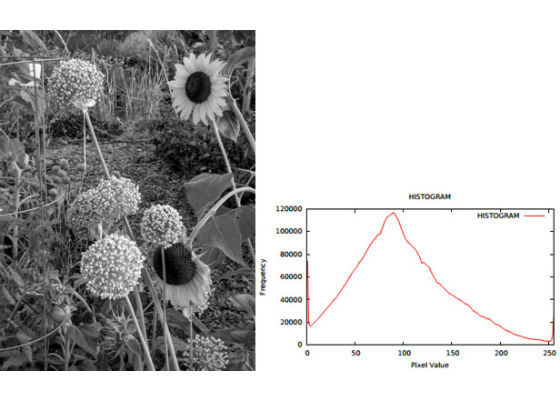Figure 1: Image used in this document (image credit: Alberto Villarreal), and its corresponding histogram.

A basic algorithm to compute the histogram is the following:

2. Get number of rows and columns in the image
3. Set image array [1: rows x columns] to image pixel values
4. Set histogram array [0: 255] to zero
5. For every pixel in the image

{
histogram [ image [ pixel ] ] = histogram [ image [ pixel ] ] + 1
}

Notice that in this basic algorithm, the image array is used as an index to the histogram array (a type conversion to an integer is assumed). This kind of indirect referencing cannot be unconditionally parallelized, because neighboring pixels in the image might have the same intensity value, in which case the results of processing more than one iteration of the loop simultaneously might be wrong.

In the next sections, this algorithm will be implemented in C++, and it will be shown that the compiler, when using the AVX-512 ISA, will be able to safely vectorize this structure (although only in a partial way, with performance depending on the image data).

It should be noticed that this implementation of a histogram computation is used in this document for pedagogical purposes only. It does not represent an efficient way to perform the histogram computation, for which there are efficient libraries available. Also, our purpose is to show, using a simple code, how the new AVX-512 ISA is adding vectorization opportunities, and to help us understand the new functionality provided by the AVX-512 ISA.

There are other ways to implement parallelism for specific examples of histogram computations. For example in (Colfax International, 2015) the authors describe a way to automatically vectorize a similar algorithm (a binning application) by modifying the code using a strip-mining technique.

Hardware

To test our application, the following system will be used:

Processor: Intel Xeon Phi processor, model 7250 (1.40 GHz)
Number of cores: 68
Number of threads: 272

The information above can be checked in a Linux* system using the command
cat /proc/cpuinfo.

Notice that when using the command shown above, the “flags” section in the output will include the “avx512f” and “avx512cd” processor flags. Those flags indicate that Intel AVX512F and Intel AVX512CD subsets are supported by this processor. Notice that the flag “avx2” is defined also, which means the Intel AVX2 ISA is also supported (although it does not take advantage of the 512-bit vector registers in this processor).

Vectorization Results Using The Intel® C++ Compiler

This section shows a basic vectorization analysis of a fragment of the histogram code. Specifically, two different loops in this code will be analyzed:

LOOP 1: A loop implementing a histogram computation only. This histogram is computed on the input image, stored in floating point single precision in array image1.

LOOP 2: A loop implementing a convolution filter followed by a histogram computation. The filter is applied to the original image in array image1 and then a new histogram is computed on the filtered image stored in array image2.
The following code section shows the two loops mentioned above (image and histogram data have been placed in aligned arrays):

// LOOP 1
#pragma vector aligned
for (position=cols; position<rows*cols-cols; position++)
{
hist1[ int(image1[position]) ]++;
}
(…)
// LOOP 2
#pragma vector aligned
for (position=cols; position<rows*cols-cols; position++)
{
if (position%cols != 0 || position%(cols-1) != 0)
{
image2[position] = ( 9.0f*image1[position]
- image1[position-1]
- image1[position+1]
- image1[position-cols-1]
- image1[position-cols+1]
- image1[position-cols]
- image1[position+cols-1]
- image1[position+cols+1]
- image1[position+cols]) ;
}
if (image2[position] >= 0 && image2[position] <= 255)
hist2[ int(image2[position]) ]++;
}

This code was compiled using Intel C++ Compiler’s option to generate an optimization report as follows:
icpc histogram.cpp -o histogram -O3 -qopt-report=2 -qopt-report-phase=vec -xCORE-AVX2  …

Note that, in this case, the -xCORE-AVX2 compiler flag has been used to ask the compiler to use the Intel AVX2 ISA to generate executable code.

The section of the optimization report that the compiler created for the loops shown above looks like this:

LOOP BEGIN at histogram.cpp(92,5)
remark #15344: loop was not vectorized: vector dependence prevents vectorization. First dependence is shown below. Use level 5 report for details
remark #15346: vector dependence: assumed FLOW dependence between  line 94 and  line 94
LOOP END
LOOP BEGIN at histogram.cpp(92,5)
<Remainder>
LOOP END
LOOP BEGIN at histogram.cpp(103,5)
remark #15344: loop was not vectorized: vector dependence prevents vectorization. First dependence is shown below. Use level 5 report for details
remark #15346: vector dependence: assumed FLOW dependence between  line 118 and  line 118
LOOP END

As can be seen in the section of the optimization report shown above, the compiler has prevented vectorization in both loops, due to dependences present in the lines of code where histogram computations are taking place (lines 94 and 118).

Now let’s compile the code using the -xMIC-AVX512 flag, to indicate the compiler to use the Intel AVX-512 ISA:
icpc histogram.cpp -o histogram -O3 -qopt-report=2 -qopt-report-phase=vec -xMIC-AVX512  …

This creates the following output for the code segment in the optimization report, showing that both loops have now been vectorized:

LOOP BEGIN at histogram.cpp(92,5)
remark #15300: LOOP WAS VECTORIZED
LOOP BEGIN at histogram.cpp(94,8)
remark #25460: No loop optimizations reported
LOOP END
LOOP END
LOOP BEGIN at histogram.cpp(92,5)
<Remainder loop for vectorization>
remark #15301: REMAINDER LOOP WAS VECTORIZED
LOOP BEGIN at histogram.cpp(94,8)
remark #25460: No loop optimizations reported
LOOP END
LOOP END
LOOP BEGIN at histogram.cpp(103,5)
remark #15300: LOOP WAS VECTORIZED
LOOP BEGIN at histogram.cpp(118,8)
remark #25460: No loop optimizations reported
LOOP END
LOOP END
LOOP BEGIN at histogram.cpp(103,5)
<Remainder loop for vectorization>
remark #15301: REMAINDER LOOP WAS VECTORIZED

The compiler reports results can be summarized as follows:

• LOOP 1, which implements a histogram computation, is not being vectorized using the Intel AVX2 flag because of an assumed dependency (which was described in section 3 in this document). However, the loop was vectorized when using the Intel AVX-512 flag, which means that the compiler has solved the dependency using instructions present in the Intel AVX-512 ISA.
• LOOP 2 gets the same diagnostics as LOOP1. The difference between these two loops is that LOOP 2 adds, on top of the histogram computation, a filter operation that has no dependencies and would be vectorizable otherwise. The presence of the histogram computation is preventing the compiler from vectorizing the entire loop (when using the Intel AVX2 flag).

Note: As can be seen in the section of the optimization report shown above, the compiler split the loop into two sections: The main loop and the reminder loop. The remainder loop contains the last few iterations in the loop (those that do not completely fill the vector unit). The compiler will usually do this, unless it knows in advance that the total number of iterations for this loop will be a multiple of the vector length.

We will ignore the reminder loop in this document. Ways to improve performance by eliminating the reminder loop are described in the literature.

Analyzing Performance of The Code

Performance of the above code segment was analyzed by adding timing instructions at the beginning and at the end of each one of the two loops, so that the time spent in each loop can be compared between different executables generated using different compiler options.

The table below shows the timing results of executing, on a single core, the vectorized and non-vectorized versions of the code (results are the average of 5 executions) using the input image without preprocessing. Baseline performance is defined here as the performance of the non-vectorized code generated by the compiler when using the Intel AVX2 compiler flag.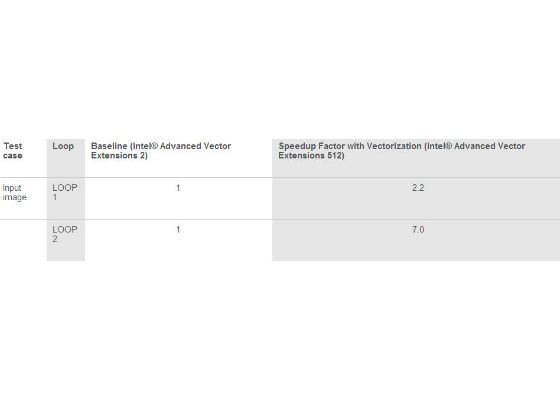To further analyze the performance of the code as a function of the input data, the input image was preprocessed using blurring and sharpening filters. Blurring filters have the effect of smoothing the image, while sharpening filters increase the contrast of the image. Blurring and sharpening filters are available in image processing or computer vision libraries. In this document, we used the OpenCV* library to preprocess the test image.

The table below shows the timing results for the three experiments: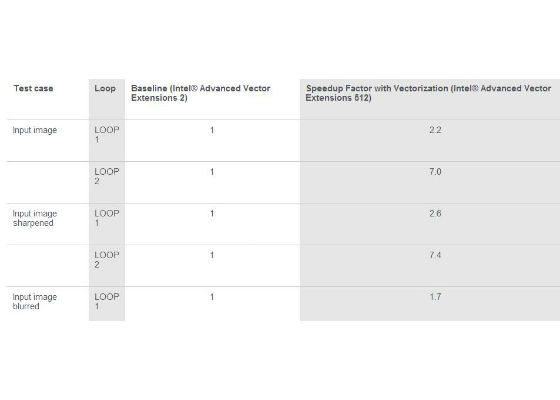Looking at the results above, three questions arise:

1. Why the compiler when using the Intel AVX512 flag is vectorizing the code, and when using the Intel AVX2 flag is not?
2. If the code in LOOP 1 using the Intel AVX512 ISA is indeed vectorized, why is the improvement in performance relatively small compared to the theoretical speedup when using 512-bit vectors?
3. Why does the performance gain of the vectorized code changes when the image is preprocessed? Specifically, why does the performance of the vectorized code increase when using a sharpened image, while it decreases when using a blurred image?

In the next section, the above questions will be answered based on a discussion about one of the subsets of the Intel AVX512 ISA, the Intel AVX512CD (conflict detection) subset.

The Intel AVX-512CD Subset

The Intel AVX-512CD (Conflict Detection) subset of the Intel AVX512 ISA adds functionality to detect data conflicts in the vector registers.

In other words, it provides functionality to detect which elements in a vector operand are identical. The result of this detection is stored in mask vectors, which are used in the vector computations, so that the histogram operation (updating the histogram array) will be performed only on elements of the array (which represent pixel values in the image) that are different.

To further explore how the new instructions from the Intel AVX512CD subset work, it is possible to ask the compiler to generate an assembly code file by using the Intel C++ Compiler –S option:

icpc example2.cpp -o example2.s -O3 -xMIC-AVX512 –S …

The above command will create, instead of the executable file, a text file containing the assembly code for our C++ source code. Let’s take a look at part of the section of the assembly code that implements line 94 (the histogram update) in LOOP 1 in the example source code:

vcvttps2dq (%r9,%rax,4), %zmm5                        #94.19 c1
vpxord    %zmm2, %zmm2, %zmm2                         #94.8 c1
kmovw     %k1, %k2                                    #94.8 c1
vpconflictd %zmm5, %zmm3                              #94.8 c3
vpgatherdd (%r12,%zmm5,4), %zmm2{%k2}                 #94.8 c3
vptestmd  %zmm0, %zmm3, %k0                           #94.8 c5
kmovw     %k0, %r10d                                  #94.8 c9 stall 1
vpaddd    %zmm1, %zmm2, %zmm4                         #94.8 c9
testl     %r10d, %r10d                                #94.8 c11
je        ..B1.165      # Prob 30%                    #94.8 c13

In the above code fragment, vpconflictd detects conflicts in the source vector register (containing the pixel values) by comparing elements with each other in the vector, and writes the results of the comparison as a bit vector to the destination. This result is further tested to define which elements in the vector register will be used simultaneously for the histogram update, using a mask vector (The vpconflictd instruction is part of the Intel AVX-512CD subset, and the vptestmd instruction is part of the Intel AVX-512F subset. Specific information about these subsets can be found in the Intel AVX-512 ISA documentation (Intel, 2016) ). This process can be described with a diagram in Figures 2 and 3.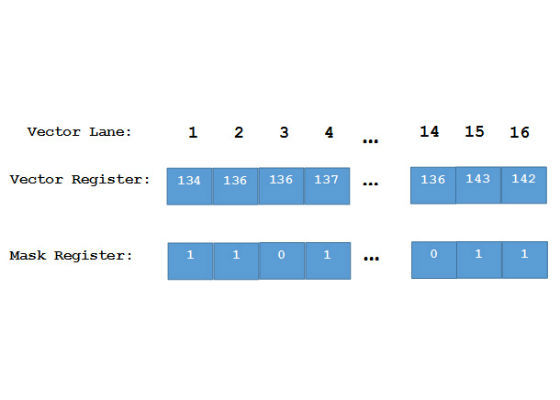Figure 2: Pixel values in array (smooth image).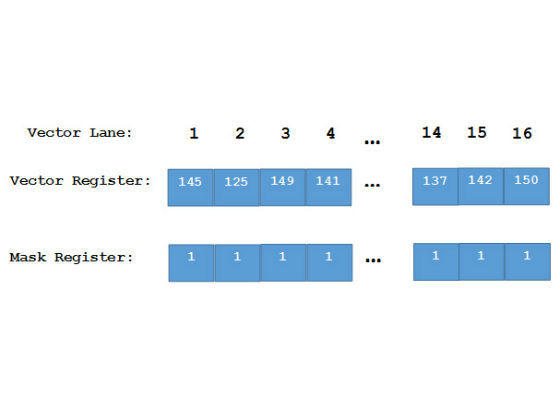Figure 3: Pixel values in array (sharp image).

Figure 2 shows the case where some neighboring pixels in the image have the same value. Only the elements in the vector register that have different values in the array image1 will be used to simultaneously update the histogram. In other words, only the elements that will not create a conflict will be used to simultaneously update the histogram. The elements in conflict will still be used to update the histogram, but at a different time.

In this case, the performance will vary depending on how smooth the image is. The worst case scenario would be when all the elements in the vector register are the same, which would decrease the performance considerably, not only because at the end the loop would be processed in scalar mode, but also because of the overhead introduced by the conflict detection and testing instructions.

Figure 3 shows the case where the image was sharpened. In this case it is more likely that neighboring pixels in the vector register will have different values. Most or all of the elements in the vector register will be used to update the histogram, thereby increasing the performance of the loop because more elements will be processed simultaneously in the vector register.

It is clear that the best performance will be obtained when all elements in the array are different. However, the best performance will still be less than the theoretical speedup (16x in this case), because of the overhead introduced by the conflict detection and testing instructions.

The above discussion can be used to get answers to the questions that arose in section 5.

Regarding the first question about why the compiler generates vectorized code when using the Intel AVX512 flag, the answer is that the Intel AVX512CD and Intel AVX512F subsets include new instructions to detect conflicts in the subsets of elements in the loop and to create conflict-free subsets that can be safely vectorized. The size of these subsets will be data dependent. Vectorization was not possible when using the Intel AVX2 flag because the Intel AVX2 ISA does not include conflict detection functionality.

The second question about the reduced performance (compared to the theoretical speedup) obtained with the vectorized code, can be answered considering that there is some overhead introduced when the conflict detection and testing instructions are executed. This penalty in performance is notorious in LOOP 1, where the only computation that takes place is the histogram update.

However, in LOOP 2, where extra work is performed (on top of the histogram update), the performance gain, relative to the baseline, increases. The compiler, using the Intel AVX512 flag, is resolving the dependency created by the histogram computation, increasing the total performance of the loop. In the Intel AVX2 case, the dependency in the histogram computation is preventing other computations in the loop (even if they are dependency-free) from running in vector mode. This is an important result of the use of the Intel AVX512CD subset. The compiler will now be able to generate vectorized code for more complex loops that include histogram-like dependencies, which possibly required code rewriting in order to be vectorized before Intel AVX-512.

To the third question, it should be noticed that the total performance of the vectorized loops becomes data-dependent when using the conflict detection mechanism. As it is shown in figures 2 and 3, the speedup when running in vector mode will depend on how many values in the vector register are not identical (conflict-free). Sharp or noisy images (in this case) are less likely to have similar/identical values in neighboring pixels, compared to a smooth/blurred image.

Conclusions

This article showed a simple example of a loop which, because of the possibility of memory conflicts, was not vectorized by the Intel C++ compiler using the Intel AVX2 (and earlier) instruction sets, but which is now vectorized when using the Intel AVX-512 ISA on an Intel Xeon Phi processor. In particular, the new functionality in the Intel AVX512CD and Intel AVX512F subsets (which is currently available in the Intel Xeon Phi processor and in future Intel Xeon processors) lets the compiler automatically generate vector code for this kind of application, with no changes to the code. However the performance of the vector code created this way will be in general less than an application running in full vector mode and will also be data dependent, because the compiler will vectorize this application using mask registers whose contents vary depending on how similar neighboring data is.

The intent in this document is to motivate the use of the new functionality in the Intel AVX-512CD and Intel AVX-512F subsets. In future documents, we will explore more possibilities for vectorization of complex loops by taking explicit control of the logic to update the mask vectors, with the purpose of increasing the efficiency of the vectorization.

For more such intel Modern Code and tools from Intel, please visit the Intel® Modern CodePromotion